Next: Measurement Up: Fundamentals of Quantum Mechanics Previous: Heisenberg's Uncertainty Principle

# Eigenstates and Eigenvalues

Consider a general real-space operator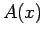. When this operator acts on a general wavefunction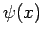the result is usually a wavefunction with a completely different shape. However, there are certain special wavefunctions which are such that whenacts on them the result is just a multiple of the original wavefunction. These special wavefunctions are called eigenstates, and the multiples are called eigenvalues. Thus, if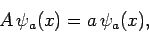(245)

whereis a complex number, then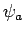is called an eigenstate ofcorresponding to the eigenvalue.

Suppose thatis an Hermitian operator corresponding to some physical dynamical variable. Consider a particle whose wavefunction is. The expectation of valuein this state is simply [see Eq. (192)](246)

where use has been made of Eq. (245) and the normalization condition (140). Moreover,(247)

so the variance ofis [cf., Eq. (160)](248)

The fact that the variance is zero implies that every measurement ofis bound to yield the same result: namely,. Thus, the eigenstateis a state which is associated with a unique value of the dynamical variable corresponding to. This unique value is simply the associated eigenvalue.

It is easily demonstrated that the eigenvalues of an Hermitian operator are all real. Recall [from Eq. (222)] that an Hermitian operator satisfies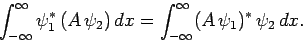(249)

Hence, if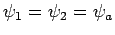then(250)

which reduces to [see Eq. (245)]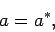(251)

assuming thatis properly normalized.

Two wavefunctions,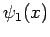and, are said to be orthogonal if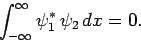(252)

Consider two eigenstates of,and, which correspond to the two different eigenvaluesand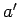, respectively. Thus,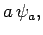(253)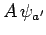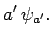(254)

Multiplying the complex conjugate of the first equation by, and the second equation by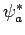, and then integrating over all, we obtain(255)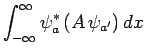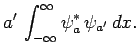(256)

However, from Eq. (249), the left-hand sides of the above two equations are equal. Hence, we can write(257)

By assumption,, yielding(258)

In other words, eigenstates of an Hermitian operator corresponding to different eigenvalues are automatically orthogonal.

Consider two eigenstates of,and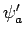, which correspond to the same eigenvalue,. Such eigenstates are termed degenerate. The above proof of the orthogonality of different eigenstates fails for degenerate eigenstates. Note, however, that any linear combination ofandis also an eigenstate ofcorresponding to the eigenvalue. Thus, even ifandare not orthogonal, we can always choose two linear combinations of these eigenstates which are orthogonal. For instance, ifandare properly normalized, and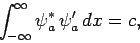(259)

then it is easily demonstrated that(260)

is a properly normalized eigenstate of, corresponding to the eigenvalue, which is orthogonal to. It is straightforward to generalize the above argument to three or more degenerate eigenstates. Hence, we conclude that the eigenstates of an Hermitian operator are, or can be chosen to be, mutually orthogonal.

It is also possible to demonstrate that the eigenstates of an Hermitian operator form a complete set: i.e., that any general wavefunction can be written as a linear combination of these eigenstates. However, the proof is quite difficult, and we shall not attempt it here.

In summary, given an Hermitian operator, any general wavefunction,, can be written(261)

where theare complex weights, and the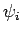are the properly normalized (and mutually orthogonal) eigenstates of: i.e.,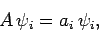(262)

whereis the eigenvalue corresponding to the eigenstate, and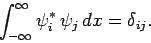(263)

Here,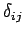is called the Kronecker delta-function, and takes the value unity when its two indices are equal, and zero otherwise.

It follows from Eqs. (261) and (263) that(264)

Thus, the expansion coefficients in Eq. (261) are easily determined, given the wavefunction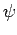and the eigenstates. Moreover, ifis a properly normalized wavefunction then Eqs. (261) and (263) yield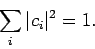(265)Next: Measurement Up: Fundamentals of Quantum Mechanics Previous: Heisenberg's Uncertainty Principle
Richard Fitzpatrick 2010-07-20